Скачать презентацию Gene-Environment Case-Control Studies Raymond J Carroll Department of

572c1b30912f43b00fd64fcab4c1d1b2.ppt

• Количество слайдов: 68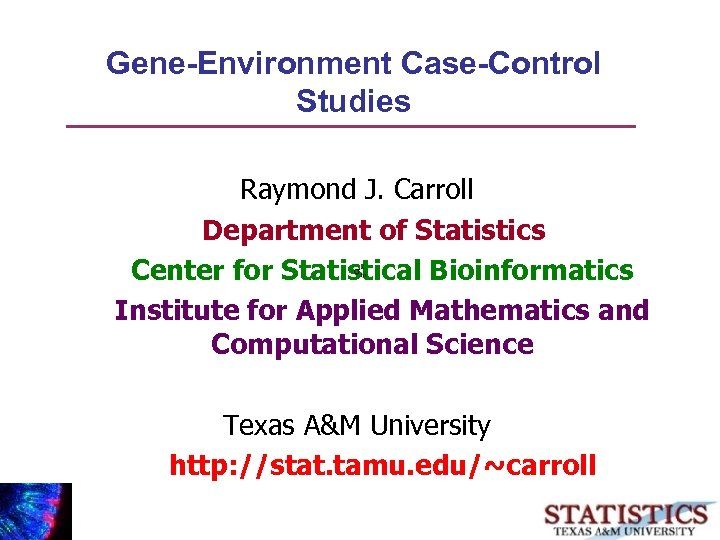Gene-Environment Case-Control Studies Raymond J. Carroll Department of Statistics Center for Statistical Bioinformatics Institute for Applied Mathematics and Computational Science Texas A&M University http: //stat. tamu. edu/~carroll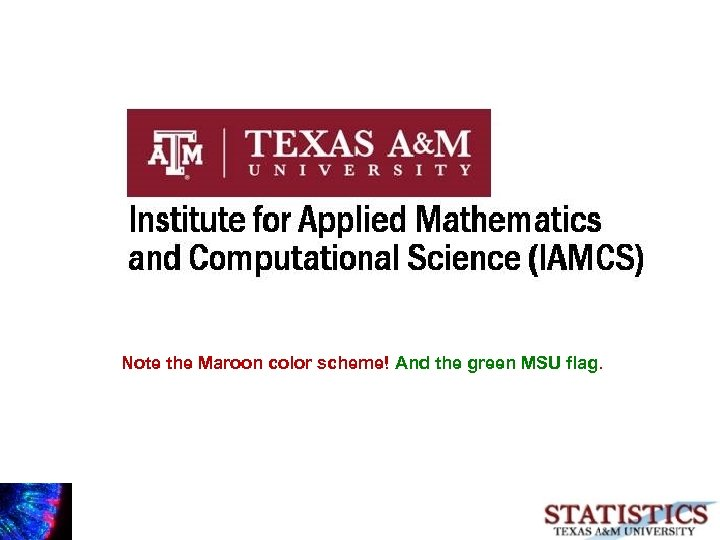Note the Maroon color scheme! And the green MSU flag.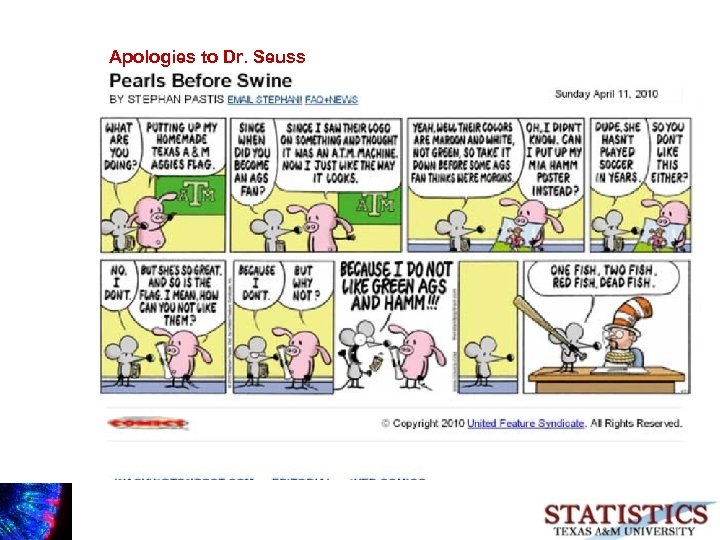Apologies to Dr. Seuss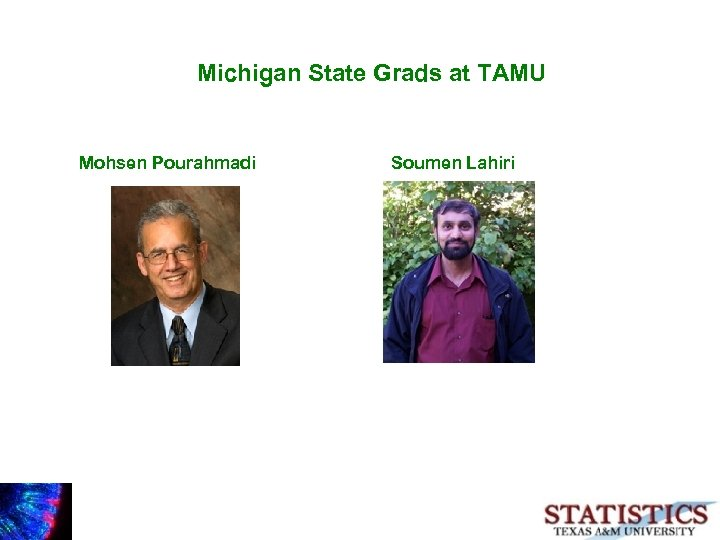Michigan State Grads at TAMU Mohsen Pourahmadi Soumen Lahiri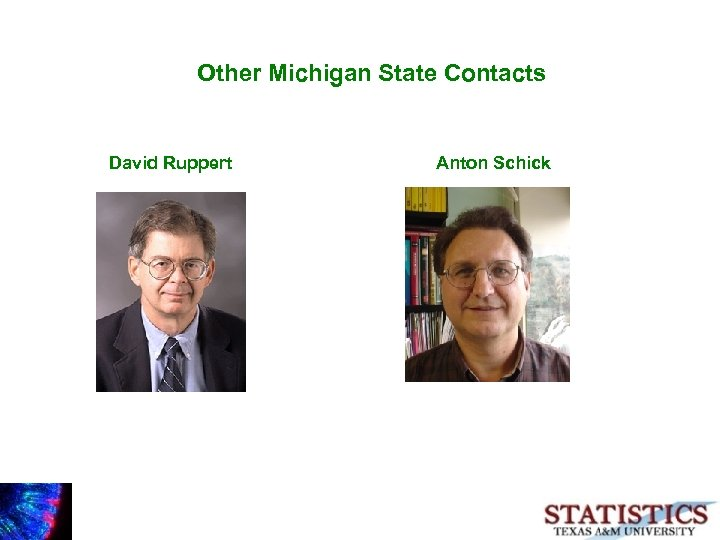Other Michigan State Contacts David Ruppert Anton Schick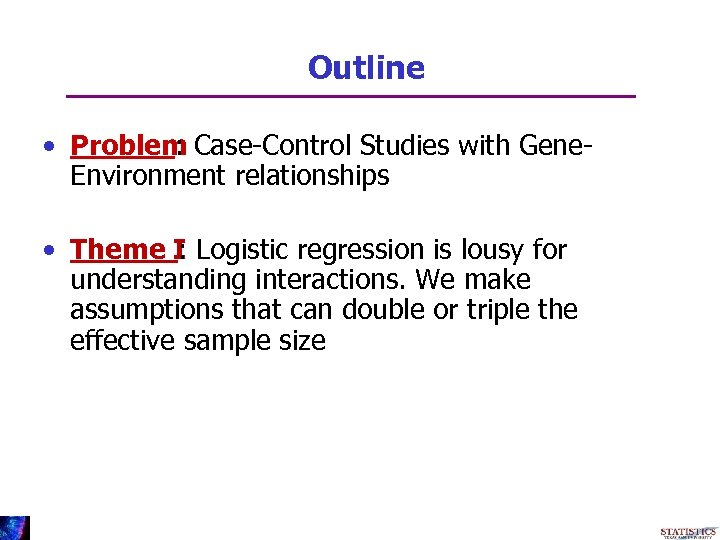Outline • Problem Case-Control Studies with Gene: Environment relationships • Theme I Logistic regression is lousy for : understanding interactions. We make assumptions that can double or triple the effective sample size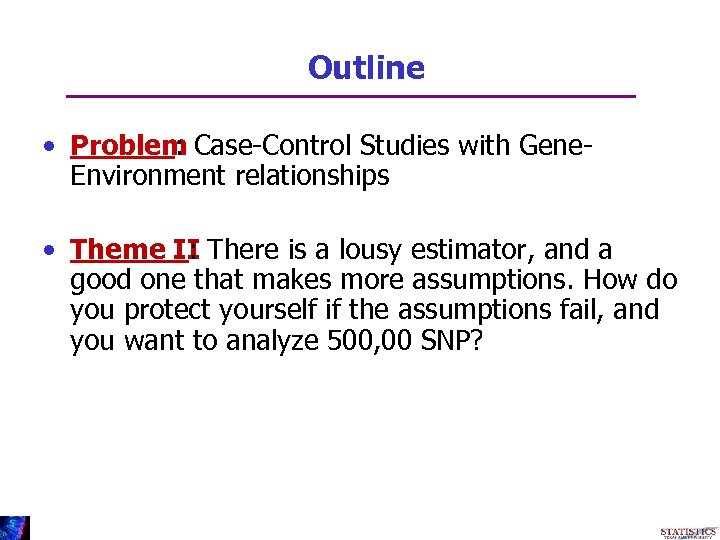Outline • Problem Case-Control Studies with Gene: Environment relationships • Theme II There is a lousy estimator, and a : good one that makes more assumptions. How do you protect yourself if the assumptions fail, and you want to analyze 500, 00 SNP?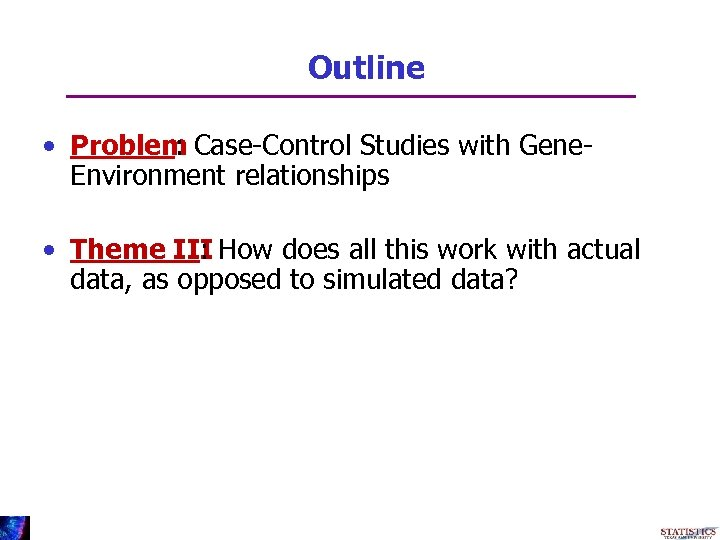Outline • Problem Case-Control Studies with Gene: Environment relationships • Theme III How does all this work with actual : data, as opposed to simulated data?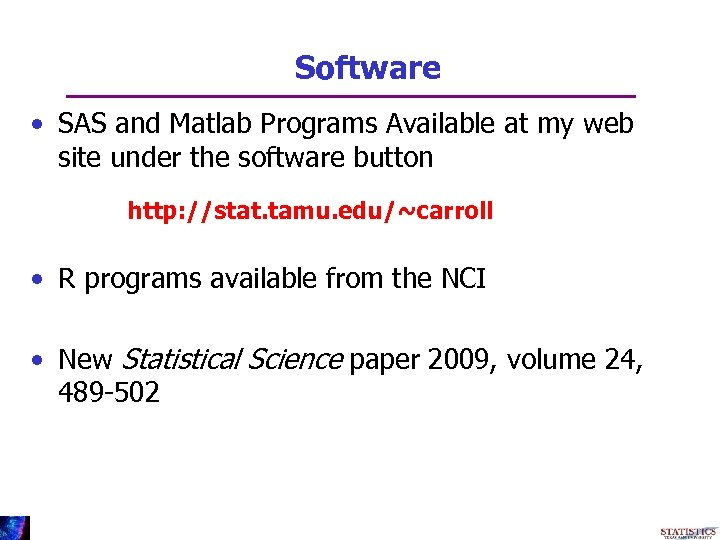Software • SAS and Matlab Programs Available at my web site under the software button http: //stat. tamu. edu/~carroll • R programs available from the NCI • New Statistical Science paper 2009, volume 24, 489 -502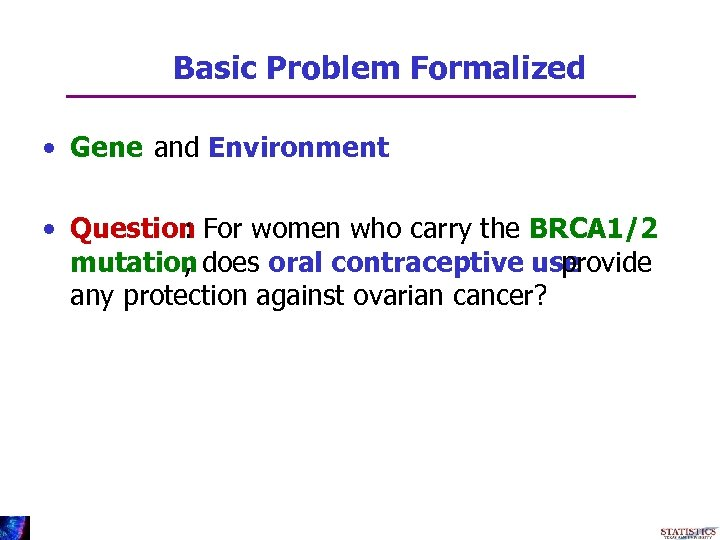Basic Problem Formalized • Gene and Environment • Question For women who carry the BRCA 1/2 : mutation does oral contraceptive use , provide any protection against ovarian cancer?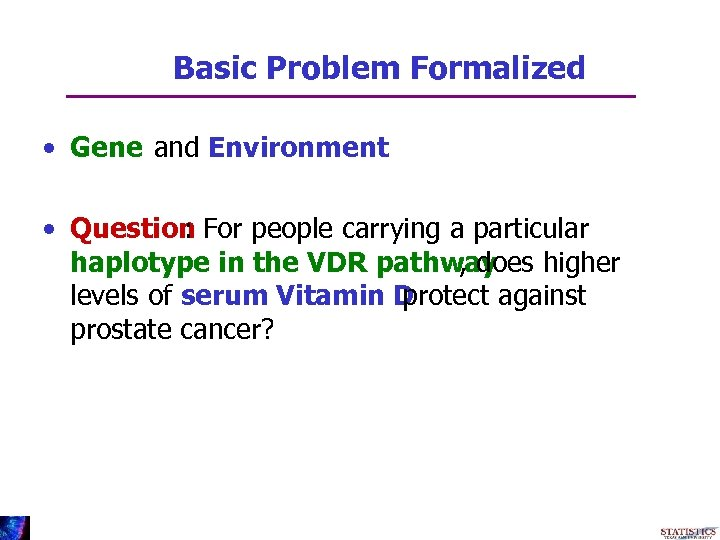Basic Problem Formalized • Gene and Environment • Question For people carrying a particular : haplotype in the VDR pathway , does higher levels of serum Vitamin D protect against prostate cancer?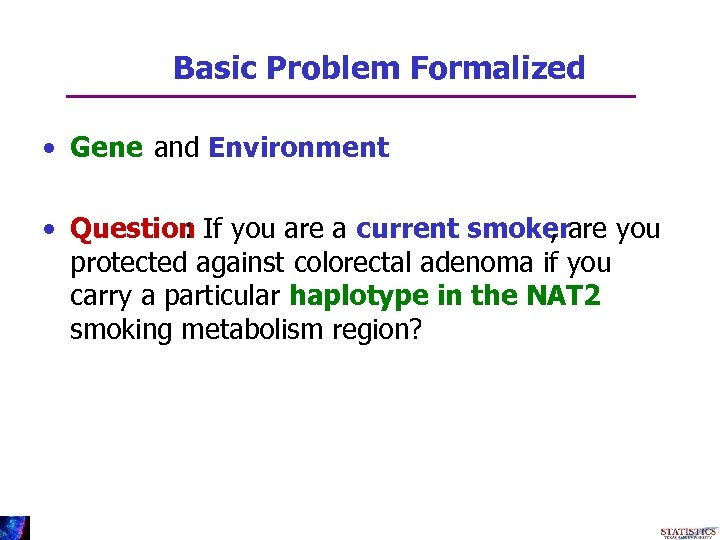Basic Problem Formalized • Gene and Environment • Question If you are a current smokerare you : , protected against colorectal adenoma if you carry a particular haplotype in the NAT 2 smoking metabolism region?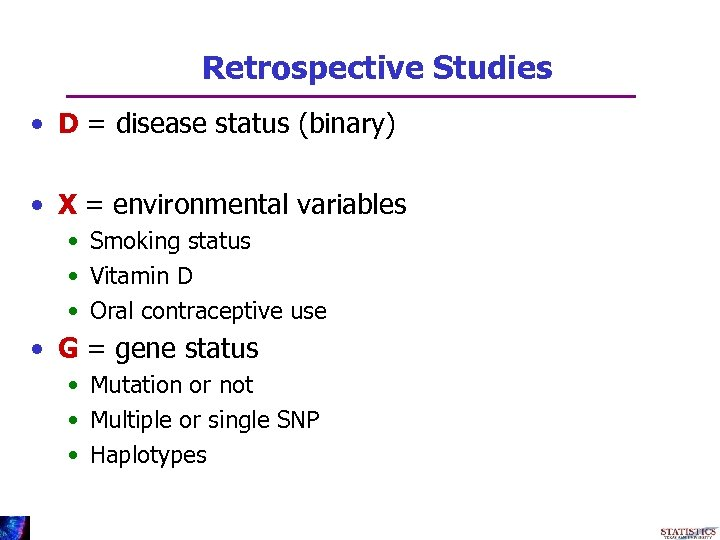Retrospective Studies • D = disease status (binary) • X = environmental variables • Smoking status • Vitamin D • Oral contraceptive use • G = gene status • Mutation or not • Multiple or single SNP • Haplotypes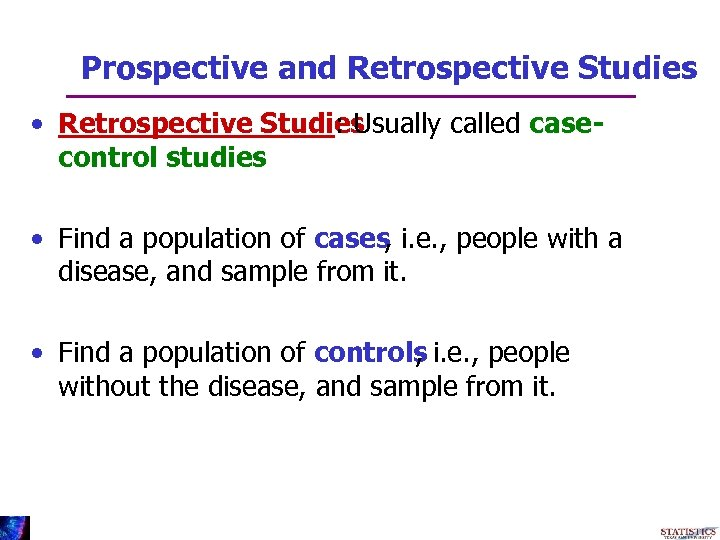Prospective and Retrospective Studies • Retrospective Studies : Usually called casecontrol studies • Find a population of cases i. e. , people with a , disease, and sample from it. • Find a population of controls i. e. , people , without the disease, and sample from it.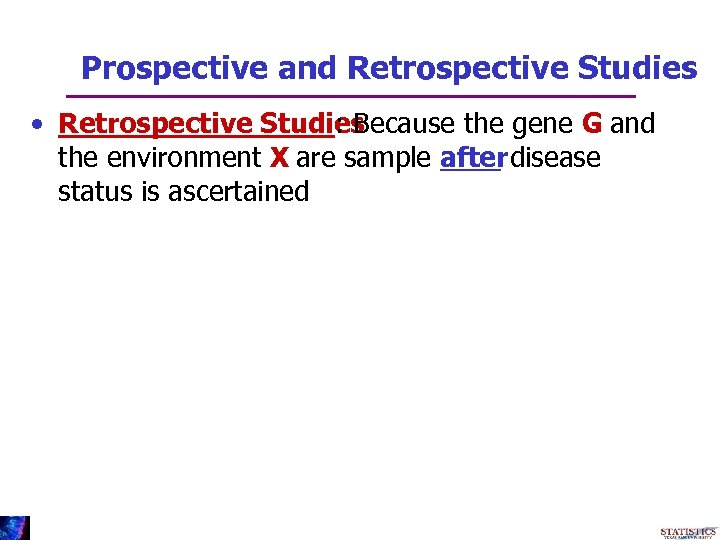Prospective and Retrospective Studies • Retrospective Studies : Because the gene G and the environment X are sample after disease status is ascertained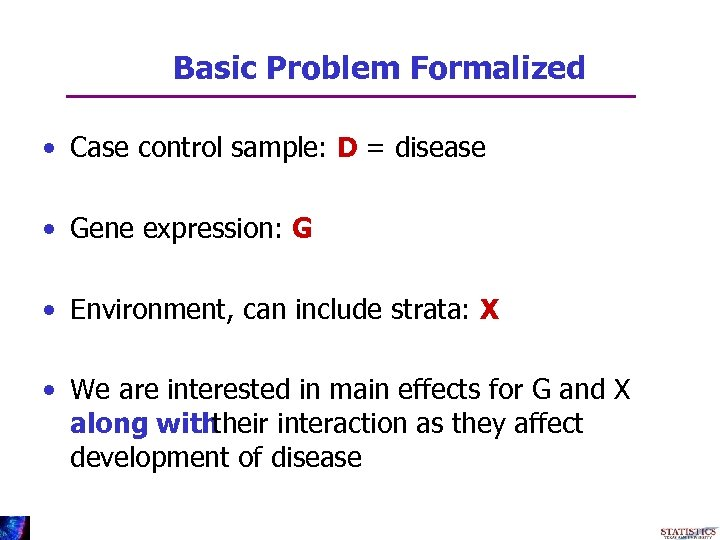Basic Problem Formalized • Case control sample: D = disease • Gene expression: G • Environment, can include strata: X • We are interested in main effects for G and X along with their interaction as they affect development of disease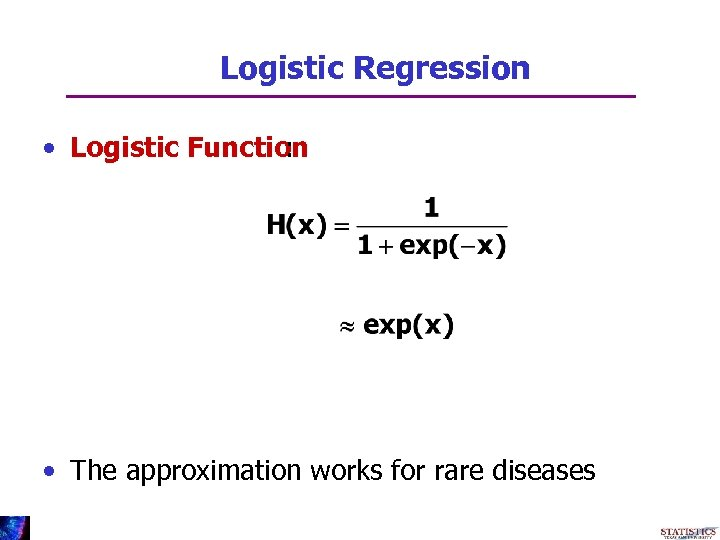Logistic Regression • Logistic Function : • The approximation works for rare diseases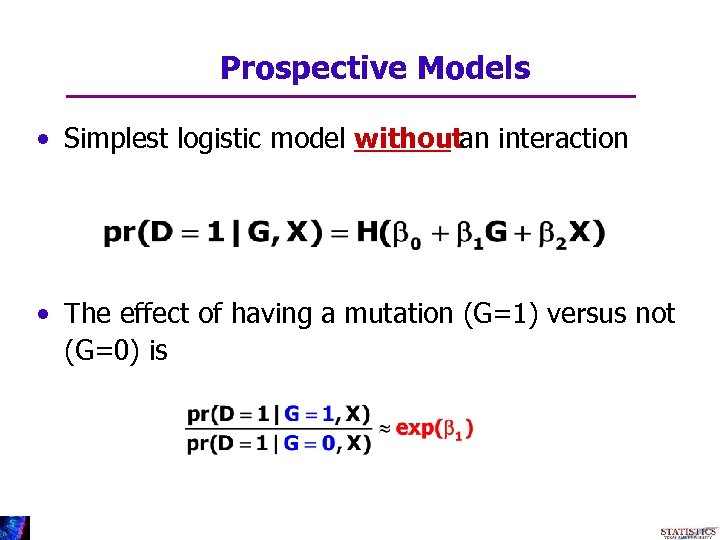Prospective Models • Simplest logistic model withoutan interaction • The effect of having a mutation (G=1) versus not (G=0) is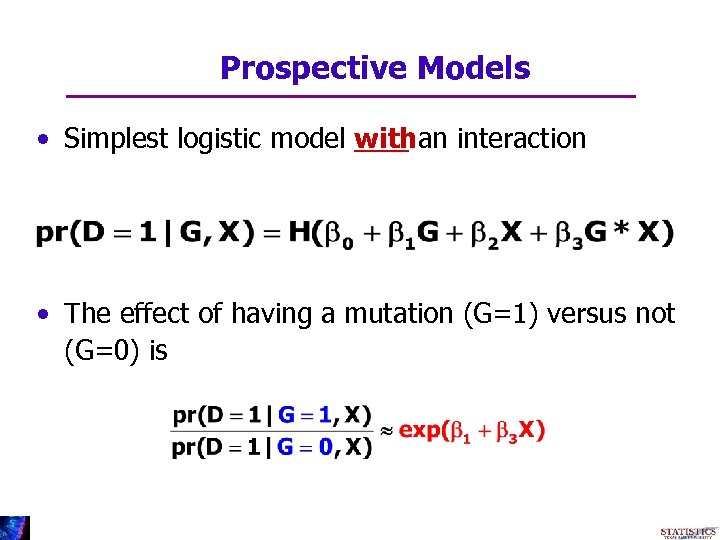Prospective Models • Simplest logistic model withan interaction • The effect of having a mutation (G=1) versus not (G=0) is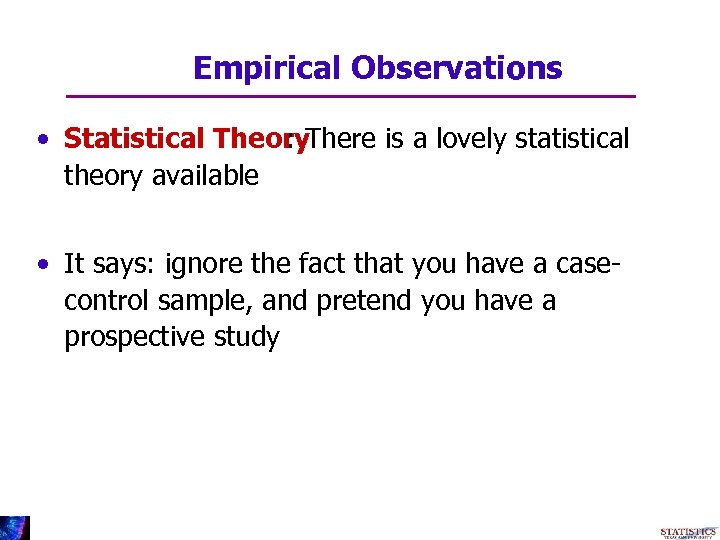Empirical Observations • Statistical Theory. There is a lovely statistical : theory available • It says: ignore the fact that you have a casecontrol sample, and pretend you have a prospective study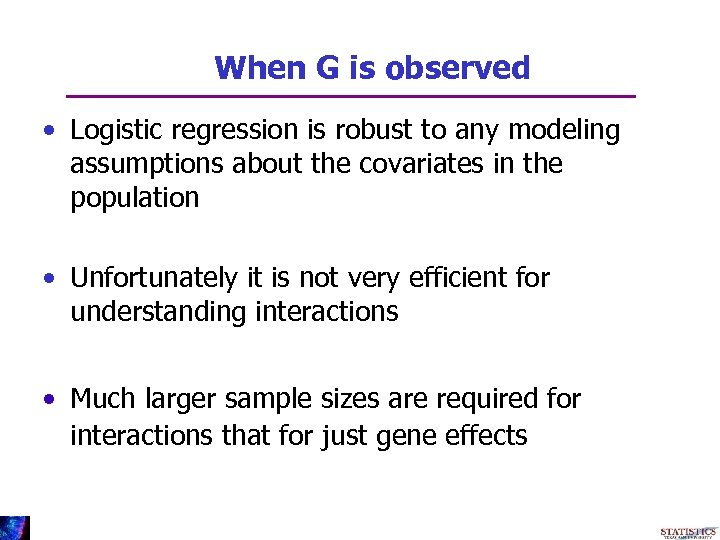When G is observed • Logistic regression is robust to any modeling assumptions about the covariates in the population • Unfortunately it is not very efficient for understanding interactions • Much larger sample sizes are required for interactions that for just gene effects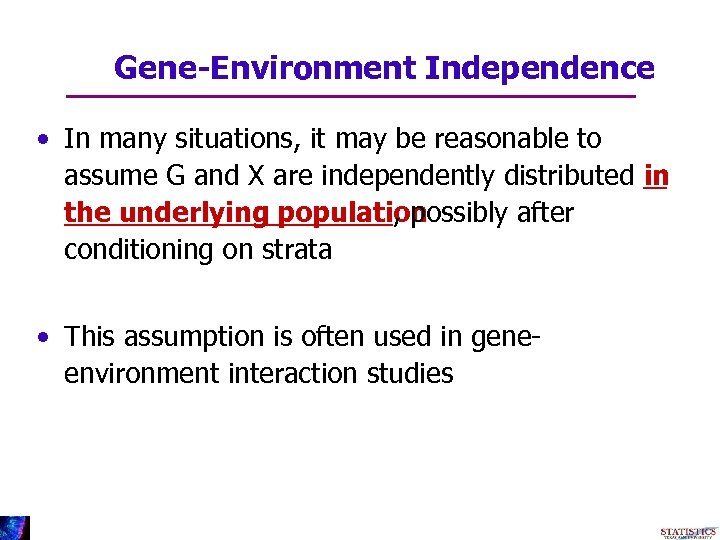Gene-Environment Independence • In many situations, it may be reasonable to assume G and X are independently distributed in the underlying population , possibly after conditioning on strata • This assumption is often used in geneenvironment interaction studies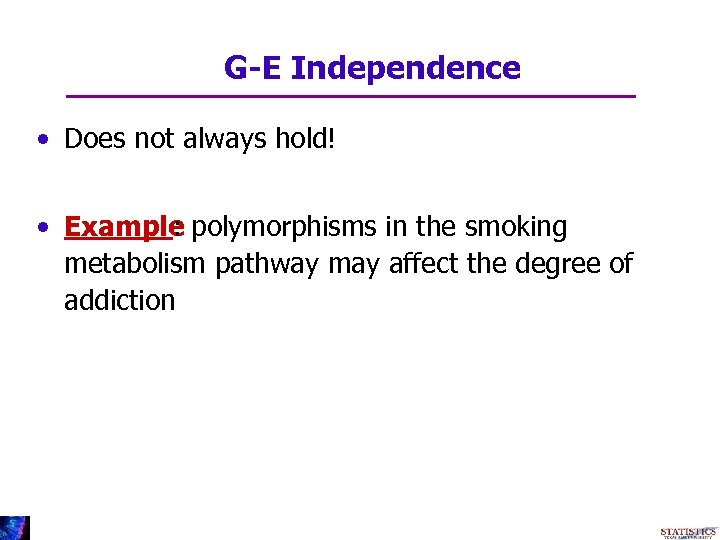G-E Independence • Does not always hold! • Example polymorphisms in the smoking : metabolism pathway may affect the degree of addiction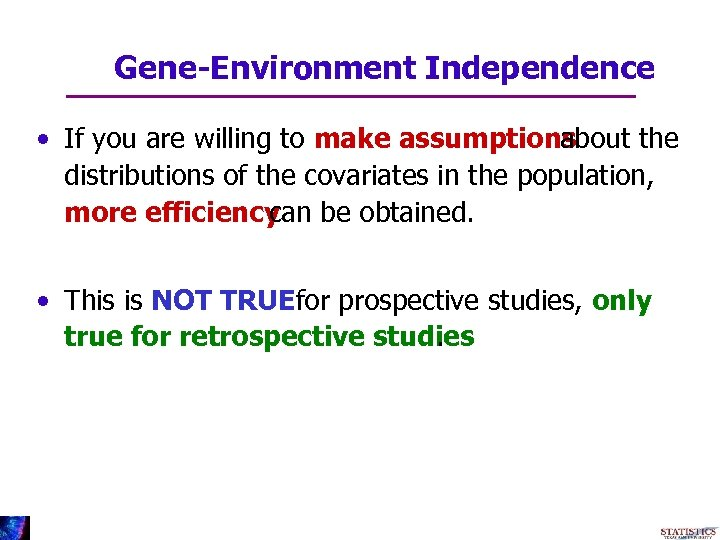Gene-Environment Independence • If you are willing to make assumptions about the distributions of the covariates in the population, more efficiency be obtained. can • This is NOT TRUEfor prospective studies, only true for retrospective studies.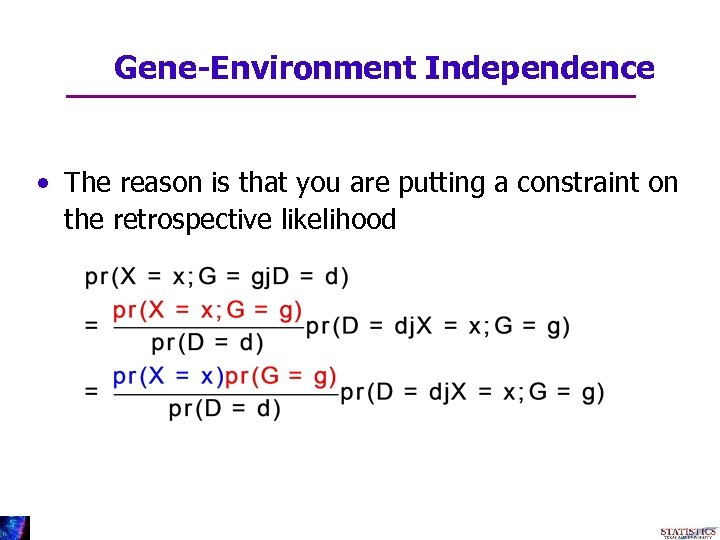Gene-Environment Independence • The reason is that you are putting a constraint on the retrospective likelihood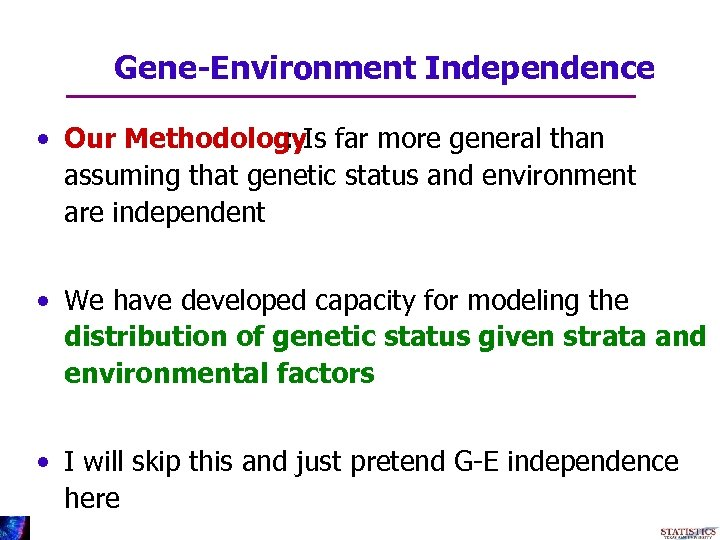Gene-Environment Independence • Our Methodology. Is far more general than : assuming that genetic status and environment are independent • We have developed capacity for modeling the distribution of genetic status given strata and environmental factors • I will skip this and just pretend G-E independence here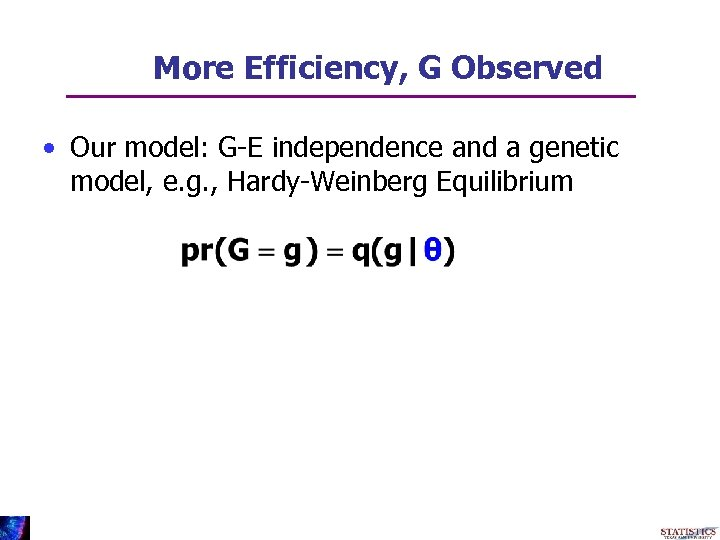More Efficiency, G Observed • Our model: G-E independence and a genetic model, e. g. , Hardy-Weinberg Equilibrium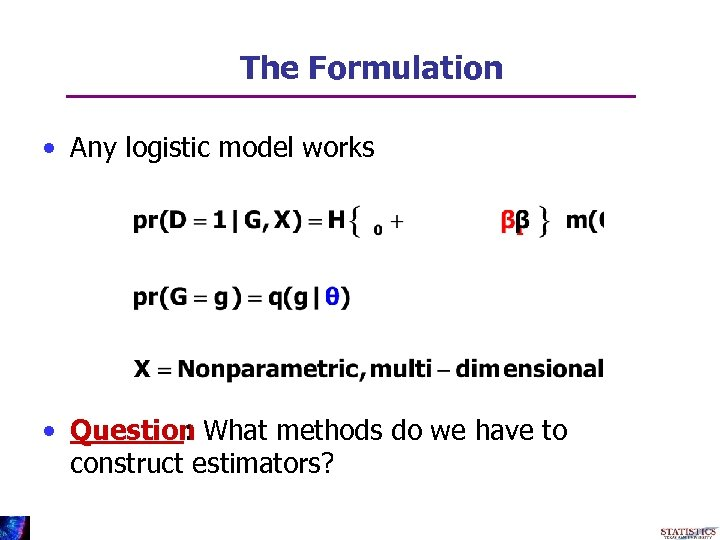The Formulation • Any logistic model works • Question What methods do we have to : construct estimators?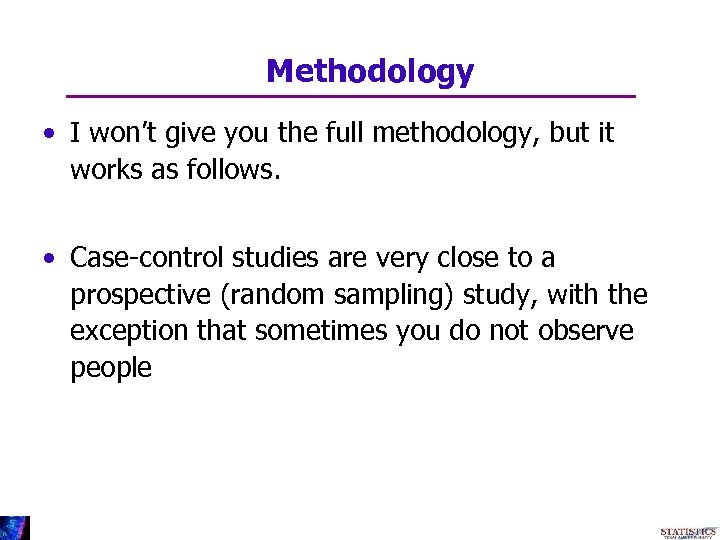Methodology • I won’t give you the full methodology, but it works as follows. • Case-control studies are very close to a prospective (random sampling) study, with the exception that sometimes you do not observe people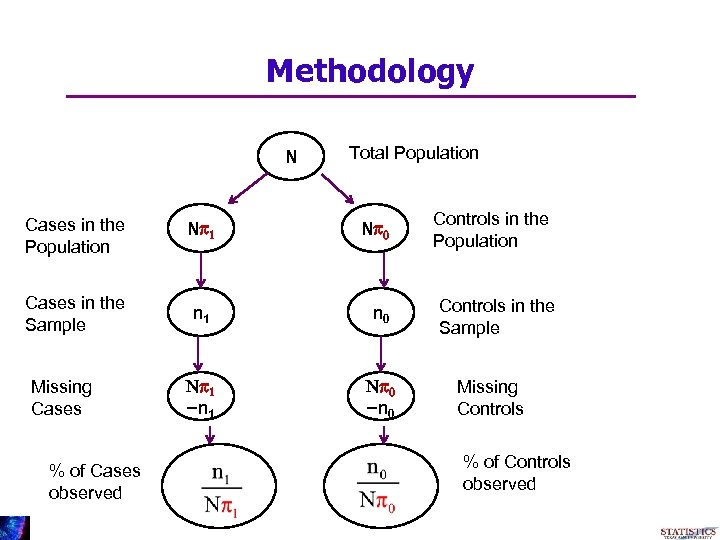Methodology N Total Population Cases in the Population Np 1 Cases in the Sample n 1 n 0 Np 1 -n 1 Np 0 -n 0 Missing Cases % of Cases observed Np 0 Controls in the Population Controls in the Sample Missing Controls % of Controls observed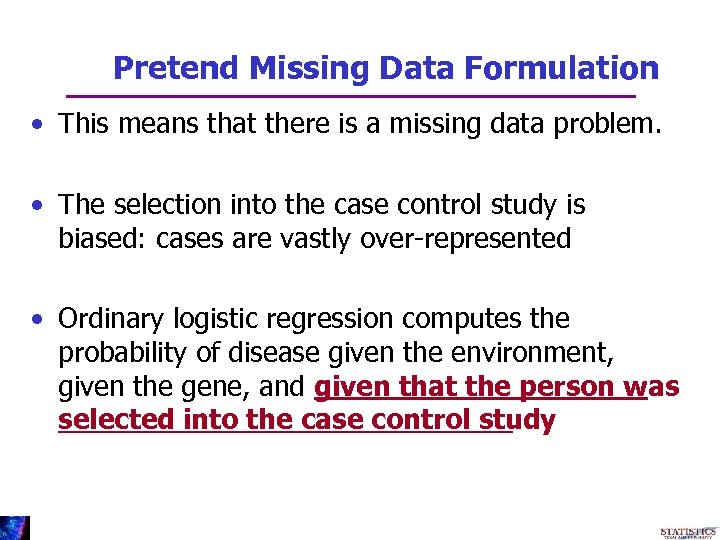Pretend Missing Data Formulation • This means that there is a missing data problem. • The selection into the case control study is biased: cases are vastly over-represented • Ordinary logistic regression computes the probability of disease given the environment, given the gene, and given that the person was selected into the case control study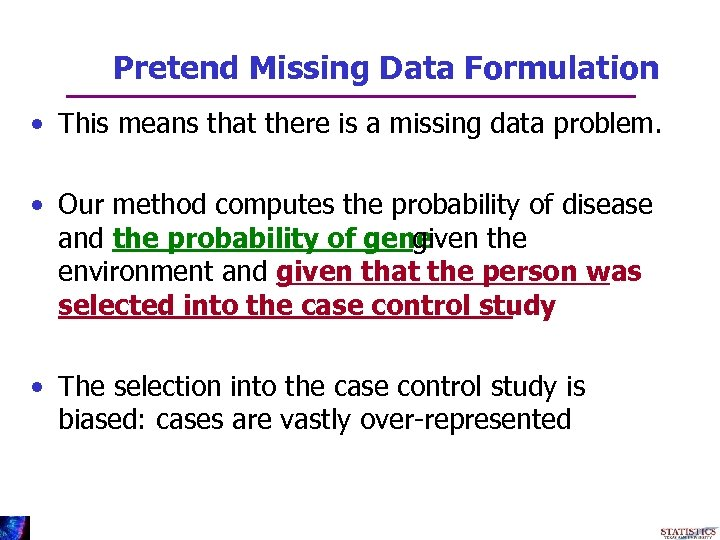Pretend Missing Data Formulation • This means that there is a missing data problem. • Our method computes the probability of disease and the probability of gene given the environment and given that the person was selected into the case control study • The selection into the case control study is biased: cases are vastly over-represented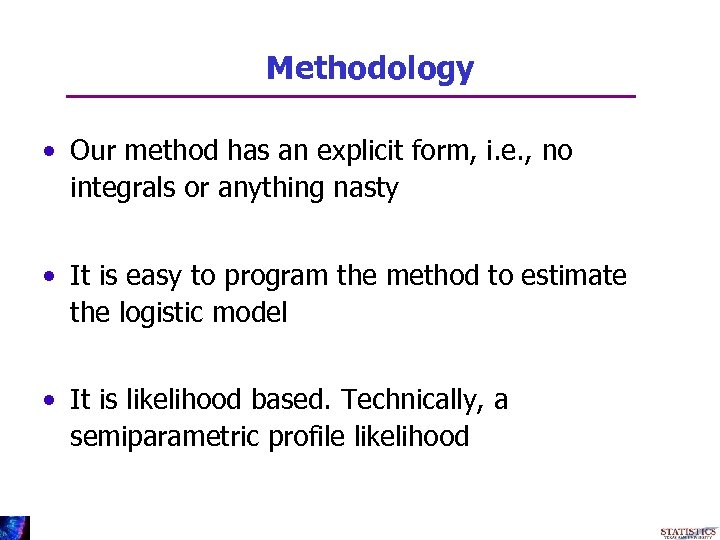Methodology • Our method has an explicit form, i. e. , no integrals or anything nasty • It is easy to program the method to estimate the logistic model • It is likelihood based. Technically, a semiparametric profile likelihood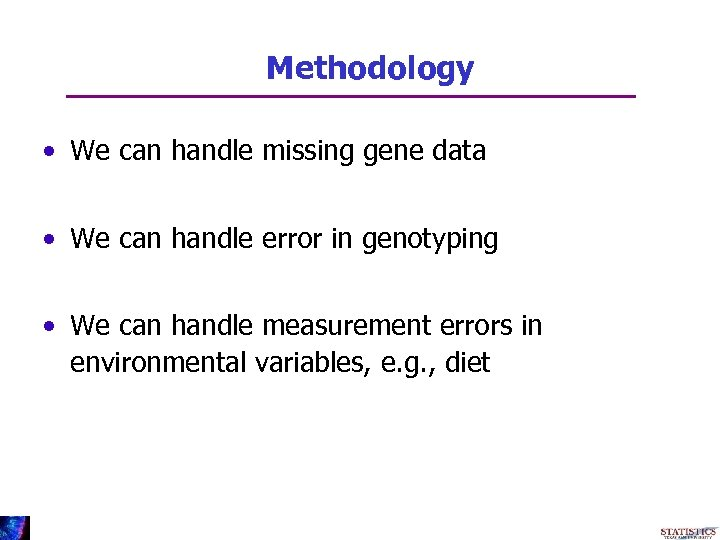Methodology • We can handle missing gene data • We can handle error in genotyping • We can handle measurement errors in environmental variables, e. g. , diet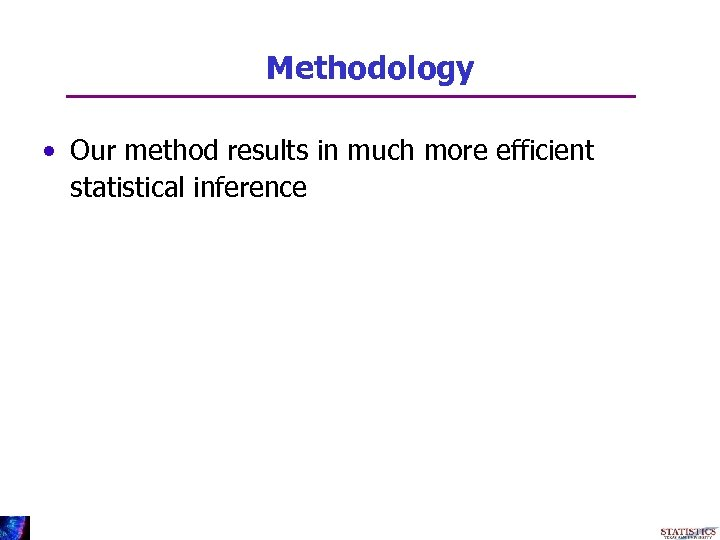Methodology • Our method results in much more efficient statistical inference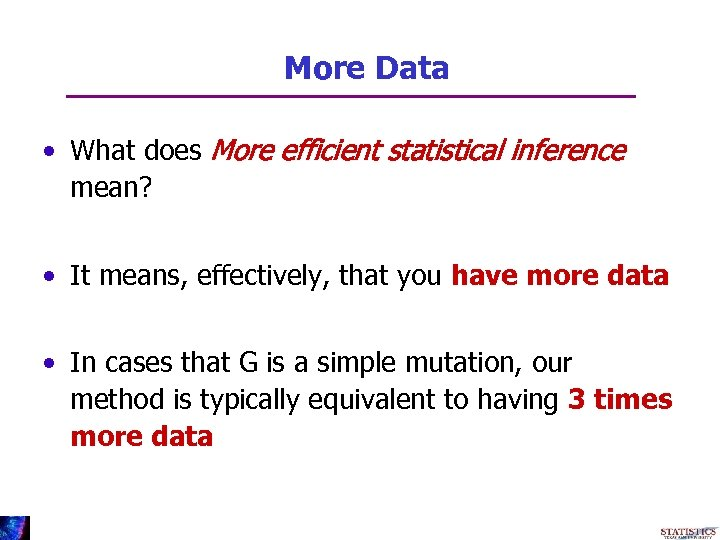More Data • What does More efficient statistical inference mean? • It means, effectively, that you have more data • In cases that G is a simple mutation, our method is typically equivalent to having 3 times more data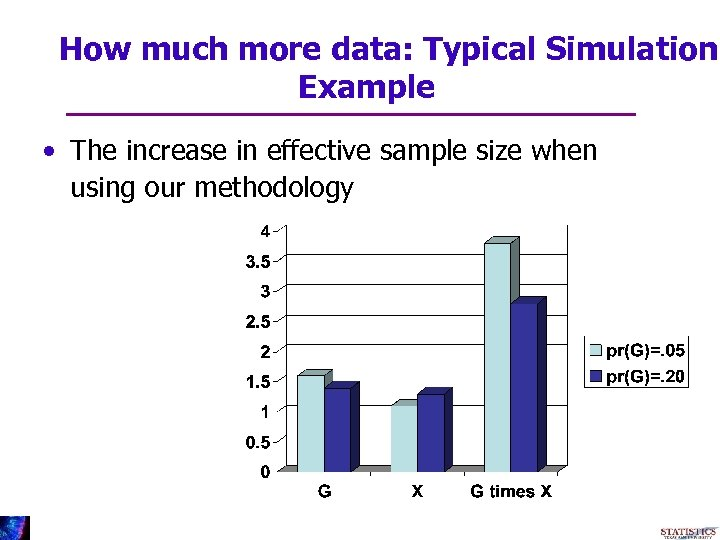How much more data: Typical Simulation Example • The increase in effective sample size when using our methodology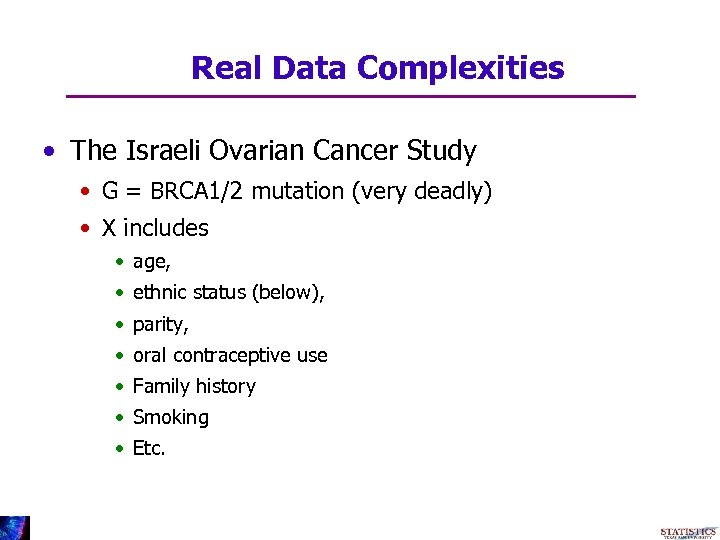Real Data Complexities • The Israeli Ovarian Cancer Study • G = BRCA 1/2 mutation (very deadly) • X includes • age, • ethnic status (below), • parity, • oral contraceptive use • Family history • Smoking • Etc.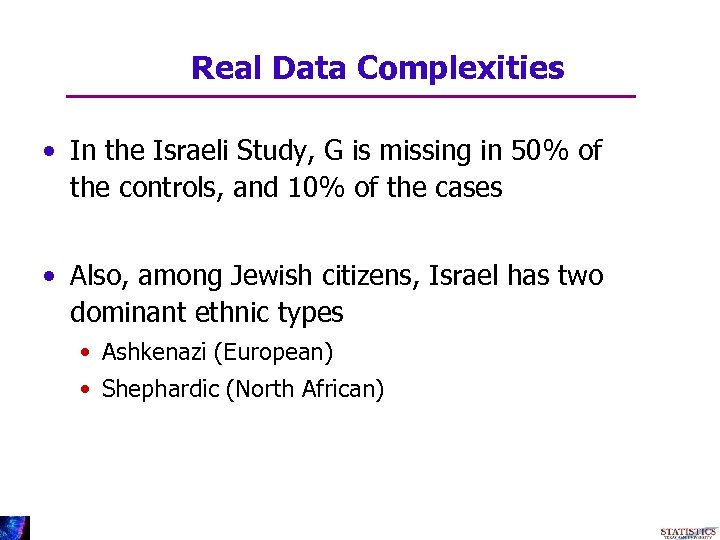Real Data Complexities • In the Israeli Study, G is missing in 50% of the controls, and 10% of the cases • Also, among Jewish citizens, Israel has two dominant ethnic types • Ashkenazi (European) • Shephardic (North African)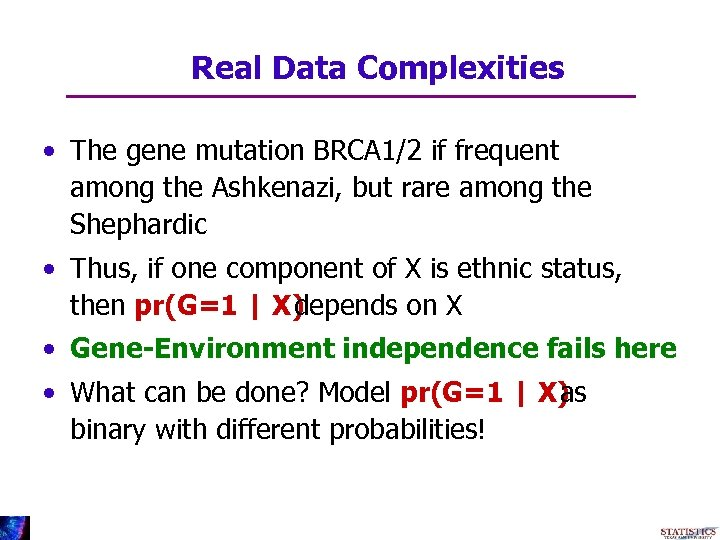Real Data Complexities • The gene mutation BRCA 1/2 if frequent among the Ashkenazi, but rare among the Shephardic • Thus, if one component of X is ethnic status, then pr(G=1 | X) depends on X • Gene-Environment independence fails here • What can be done? Model pr(G=1 | X) as binary with different probabilities!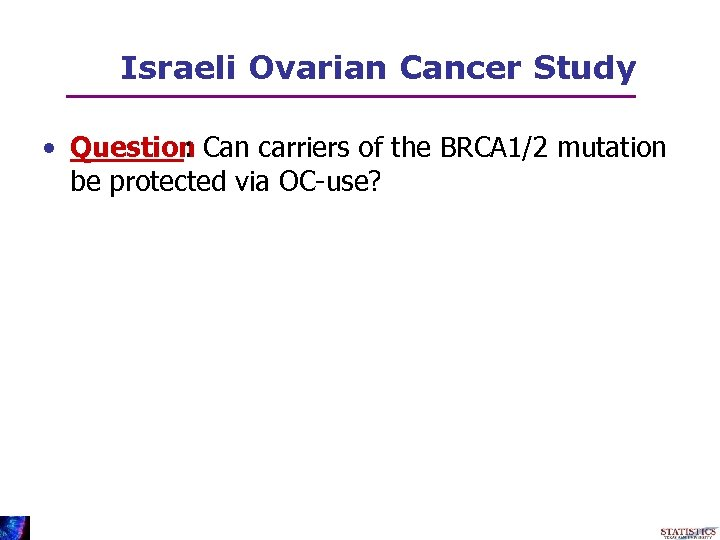Israeli Ovarian Cancer Study • Question Can carriers of the BRCA 1/2 mutation : be protected via OC-use?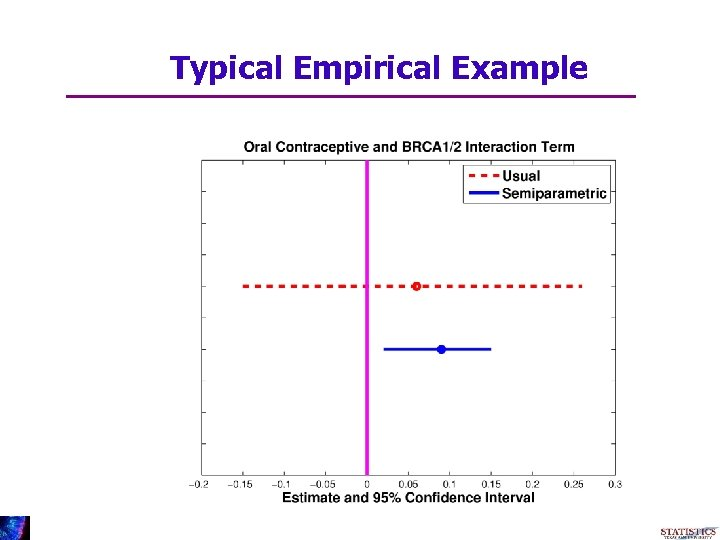Typical Empirical Example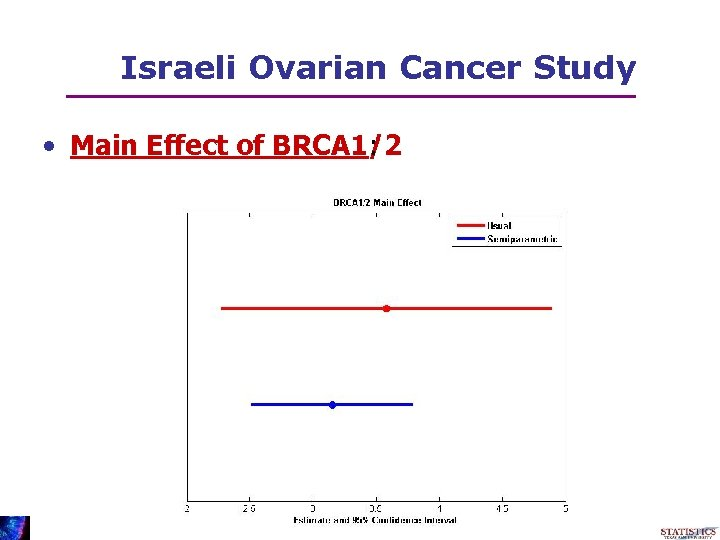Israeli Ovarian Cancer Study • Main Effect of BRCA 1/2 :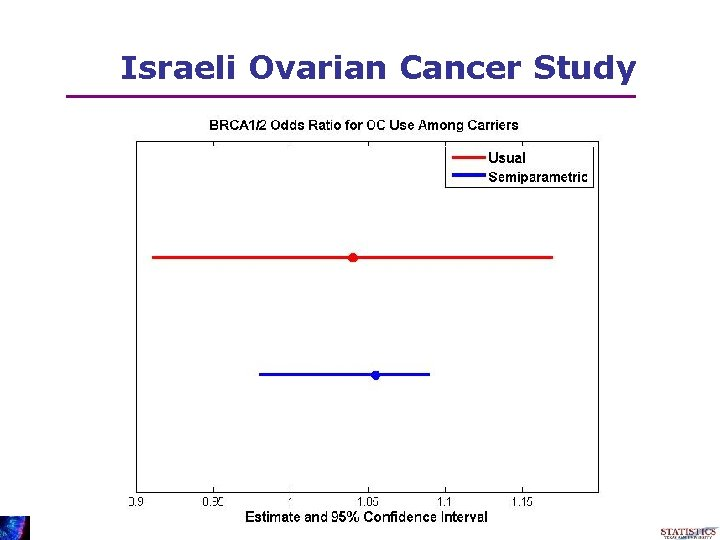Israeli Ovarian Cancer Study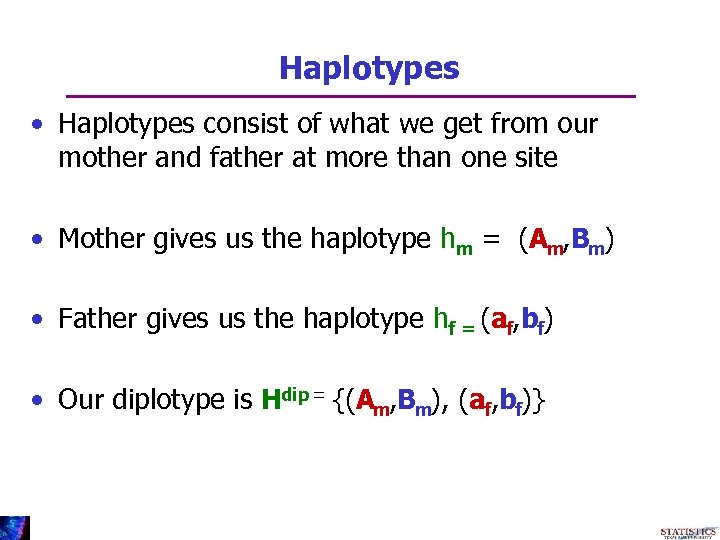Haplotypes • Haplotypes consist of what we get from our mother and father at more than one site • Mother gives us the haplotype hm = (Am, Bm) • Father gives us the haplotype hf = (af, bf) • Our diplotype is Hdip = {(Am, Bm), (af, bf)}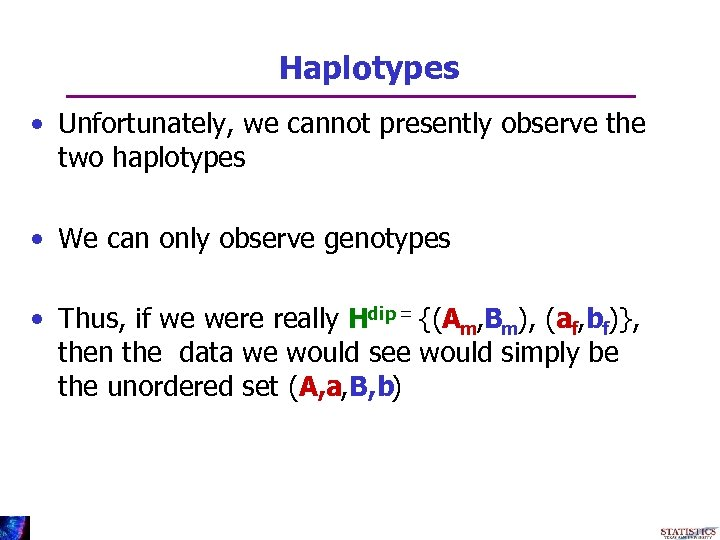Haplotypes • Unfortunately, we cannot presently observe the two haplotypes • We can only observe genotypes • Thus, if we were really Hdip = {(Am, Bm), (af, bf)}, then the data we would see would simply be the unordered set (A, a, B, b)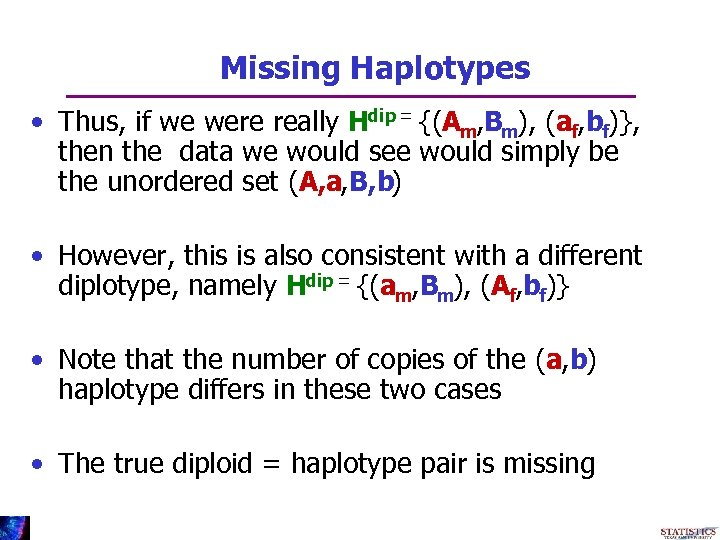Missing Haplotypes • Thus, if we were really Hdip = {(Am, Bm), (af, bf)}, then the data we would see would simply be the unordered set (A, a, B, b) • However, this is also consistent with a different diplotype, namely Hdip = {(am, Bm), (Af, bf)} • Note that the number of copies of the (a, b) haplotype differs in these two cases • The true diploid = haplotype pair is missing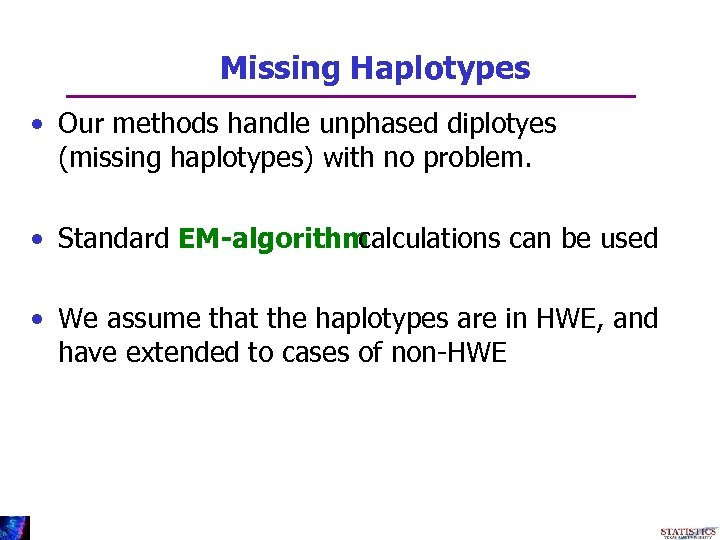Missing Haplotypes • Our methods handle unphased diplotyes (missing haplotypes) with no problem. • Standard EM-algorithm calculations can be used • We assume that the haplotypes are in HWE, and have extended to cases of non-HWE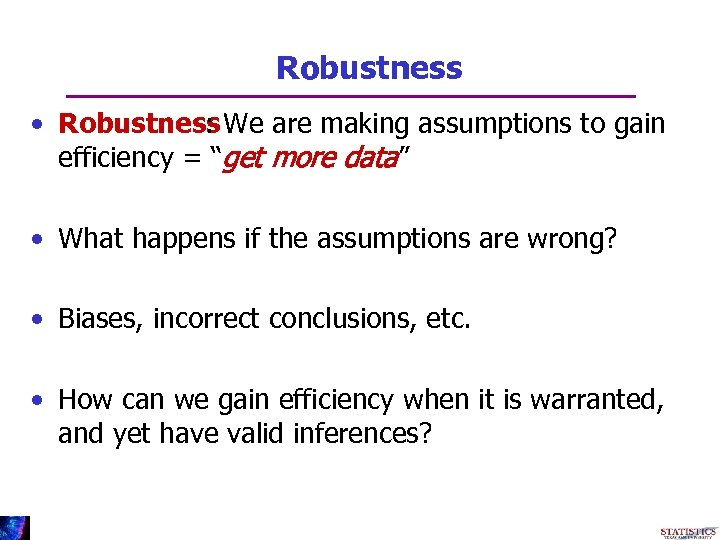Robustness • Robustness We are making assumptions to gain : efficiency = “get more data” • What happens if the assumptions are wrong? • Biases, incorrect conclusions, etc. • How can we gain efficiency when it is warranted, and yet have valid inferences?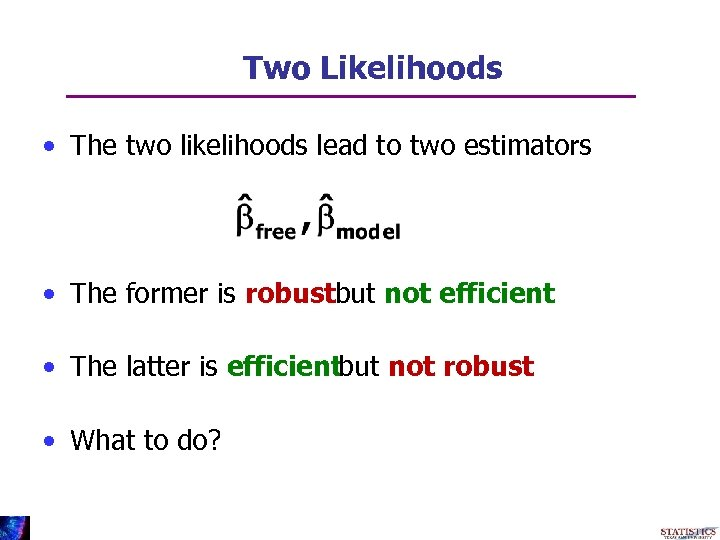Two Likelihoods • The two likelihoods lead to two estimators • The former is robustbut not efficient • The latter is efficientbut not robust • What to do?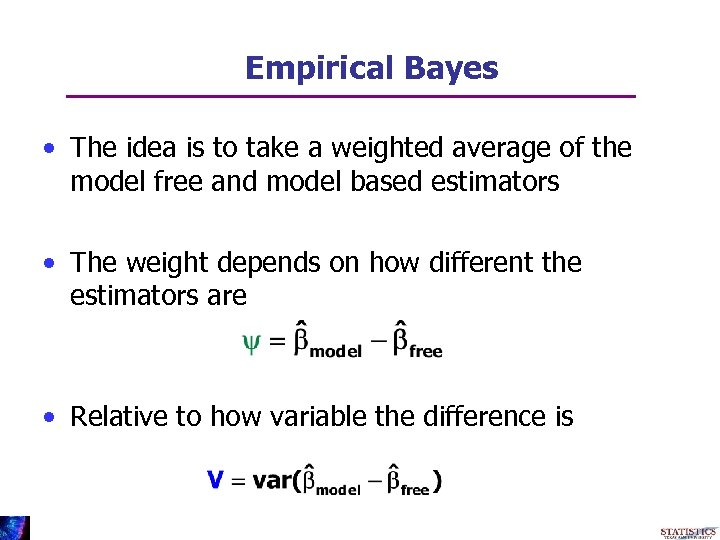Empirical Bayes • The idea is to take a weighted average of the model free and model based estimators • The weight depends on how different the estimators are • Relative to how variable the difference is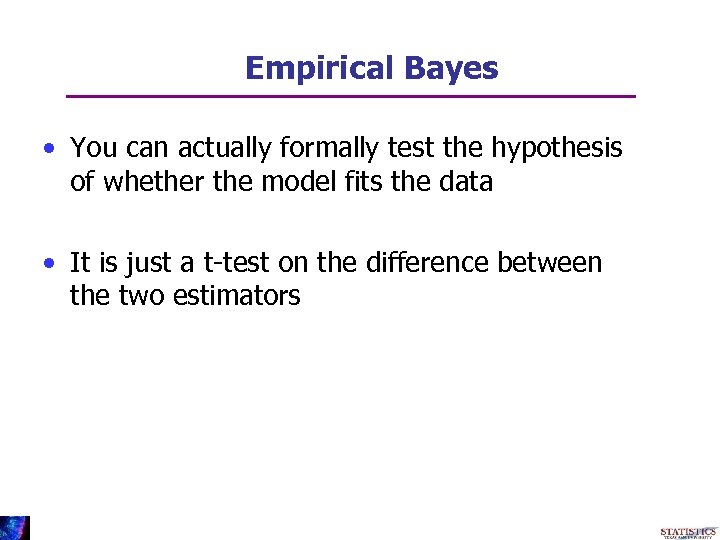Empirical Bayes • You can actually formally test the hypothesis of whether the model fits the data • It is just a t-test on the difference between the two estimators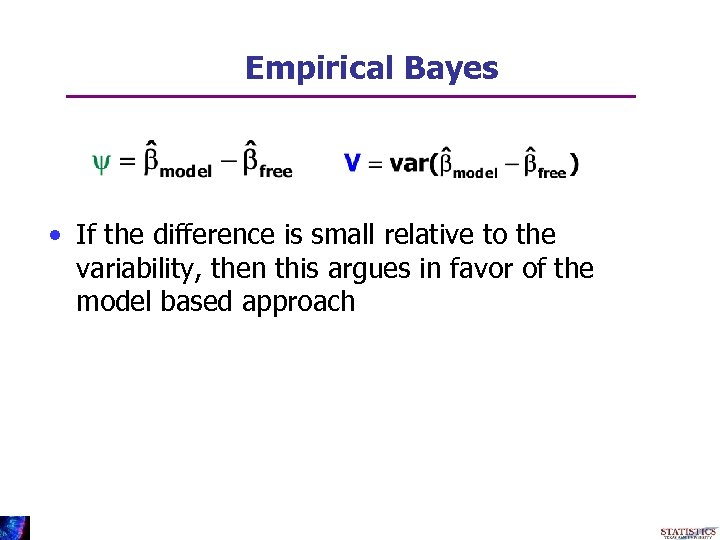Empirical Bayes • If the difference is small relative to the variability, then this argues in favor of the model based approach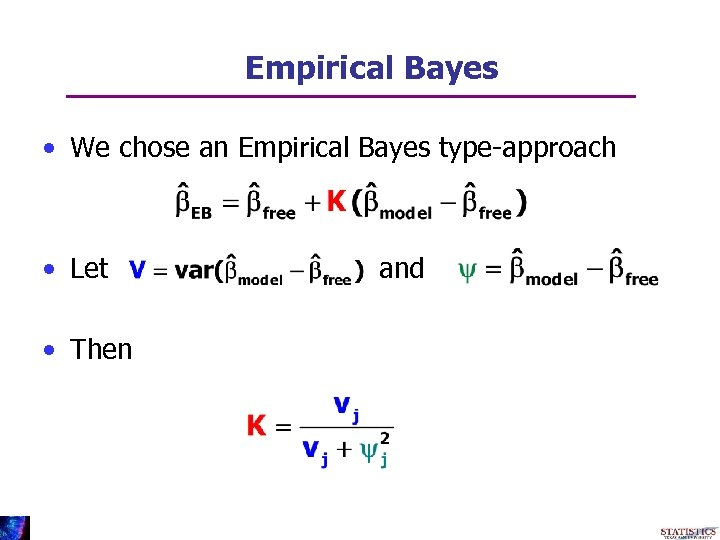Empirical Bayes • We chose an Empirical Bayes type-approach • Let • Then and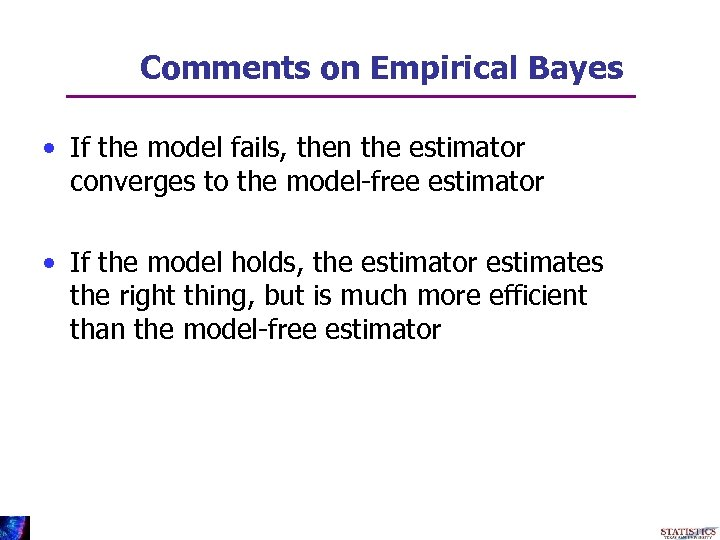Comments on Empirical Bayes • If the model fails, then the estimator converges to the model-free estimator • If the model holds, the estimator estimates the right thing, but is much more efficient than the model-free estimator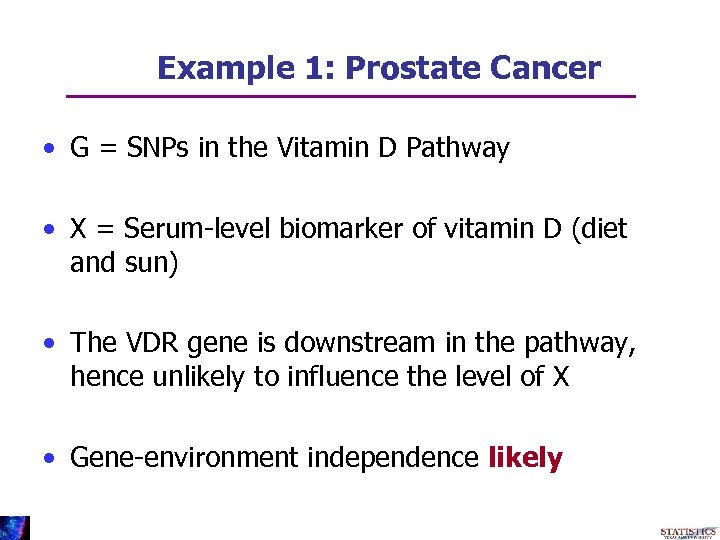Example 1: Prostate Cancer • G = SNPs in the Vitamin D Pathway • X = Serum-level biomarker of vitamin D (diet and sun) • The VDR gene is downstream in the pathway, hence unlikely to influence the level of X • Gene-environment independence likely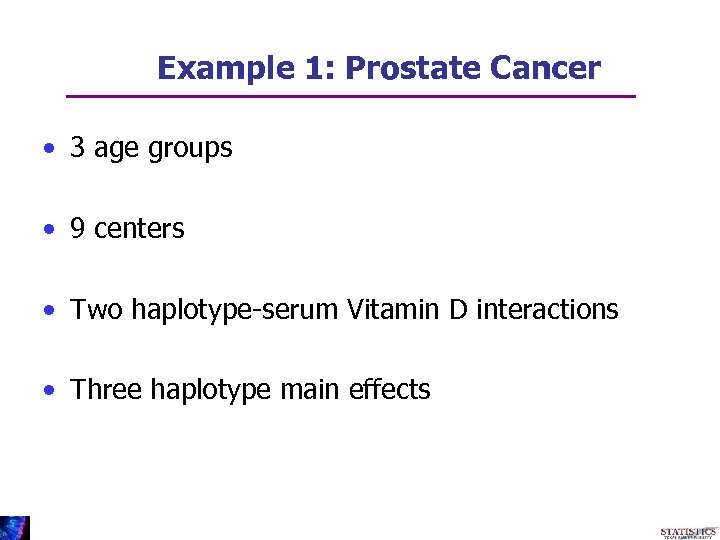Example 1: Prostate Cancer • 3 age groups • 9 centers • Two haplotype-serum Vitamin D interactions • Three haplotype main effects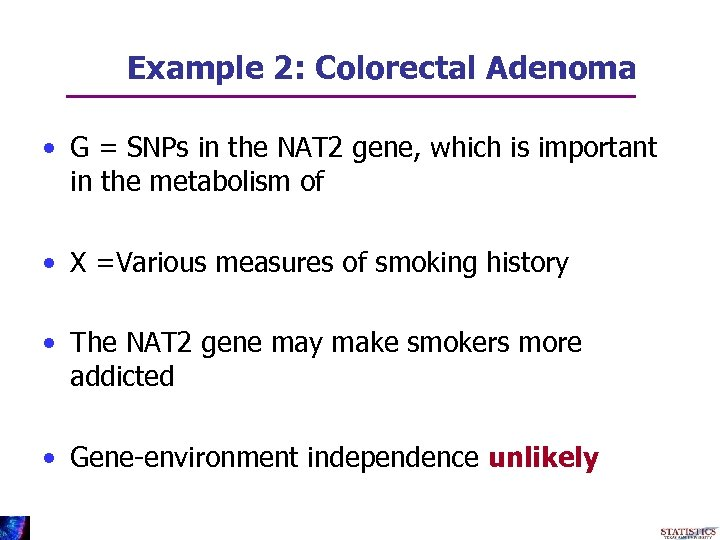Example 2: Colorectal Adenoma • G = SNPs in the NAT 2 gene, which is important in the metabolism of • X =Various measures of smoking history • The NAT 2 gene may make smokers more addicted • Gene-environment independence unlikely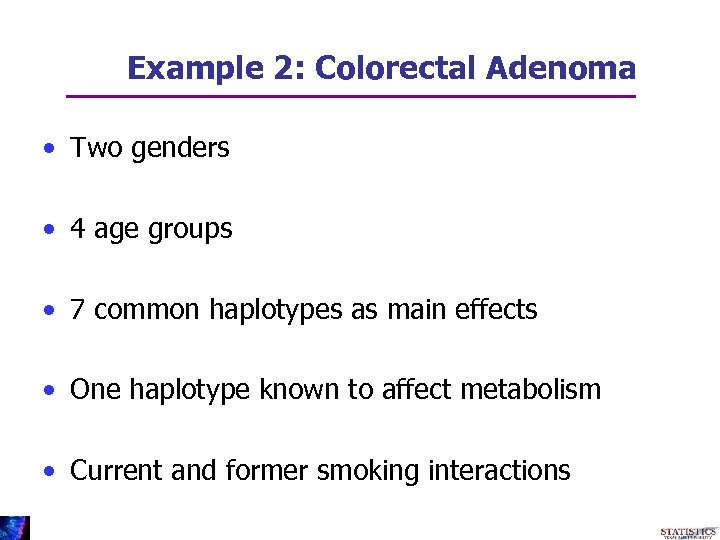Example 2: Colorectal Adenoma • Two genders • 4 age groups • 7 common haplotypes as main effects • One haplotype known to affect metabolism • Current and former smoking interactions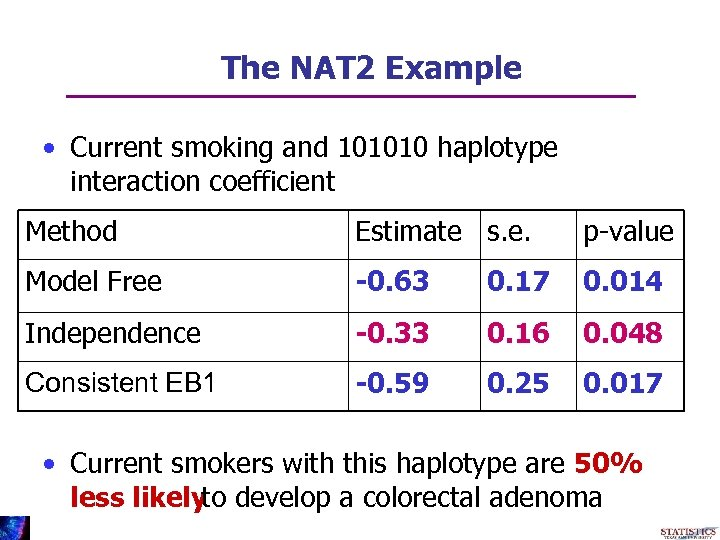The NAT 2 Example • Current smoking and 101010 haplotype interaction coefficient Method Estimate s. e. p-value Model Free -0. 63 0. 17 0. 014 Independence -0. 33 0. 16 0. 048 Consistent EB 1 -0. 59 0. 25 0. 017 • Current smokers with this haplotype are 50% less likely develop a colorectal adenoma to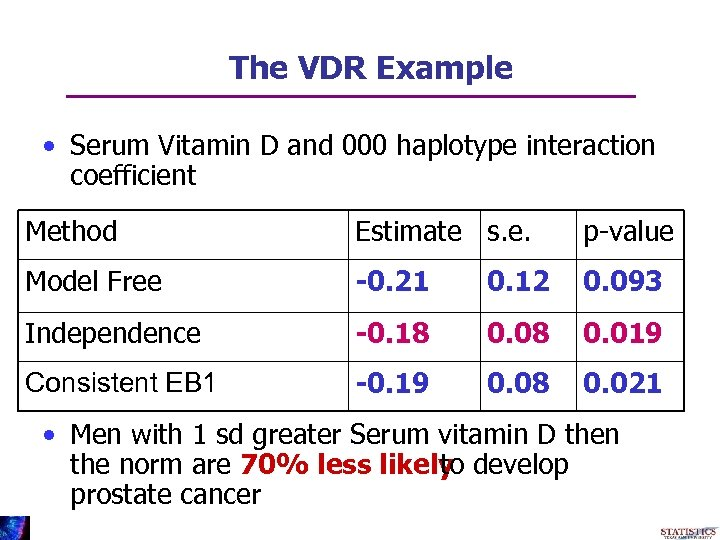The VDR Example • Serum Vitamin D and 000 haplotype interaction coefficient Method Estimate s. e. p-value Model Free -0. 21 0. 12 0. 093 Independence -0. 18 0. 019 Consistent EB 1 -0. 19 0. 08 0. 021 • Men with 1 sd greater Serum vitamin D then the norm are 70% less likely develop to prostate cancer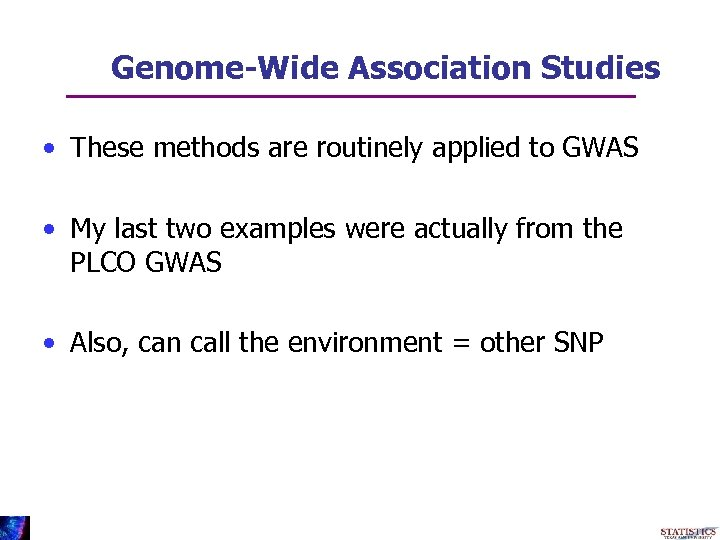Genome-Wide Association Studies • These methods are routinely applied to GWAS • My last two examples were actually from the PLCO GWAS • Also, can call the environment = other SNP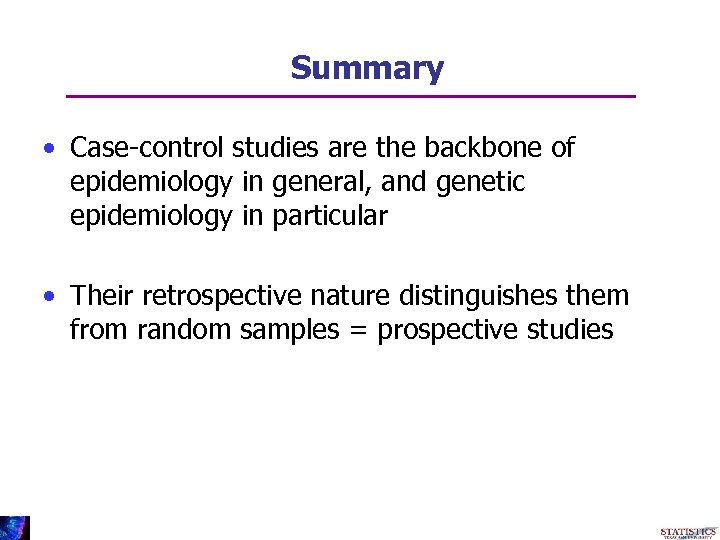Summary • Case-control studies are the backbone of epidemiology in general, and genetic epidemiology in particular • Their retrospective nature distinguishes them from random samples = prospective studies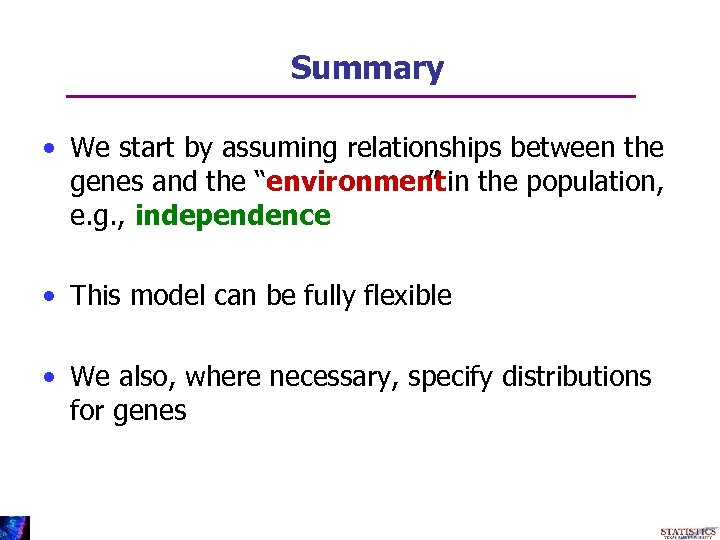Summary • We start by assuming relationships between the genes and the “environment in the population, ” e. g. , independence • This model can be fully flexible • We also, where necessary, specify distributions for genes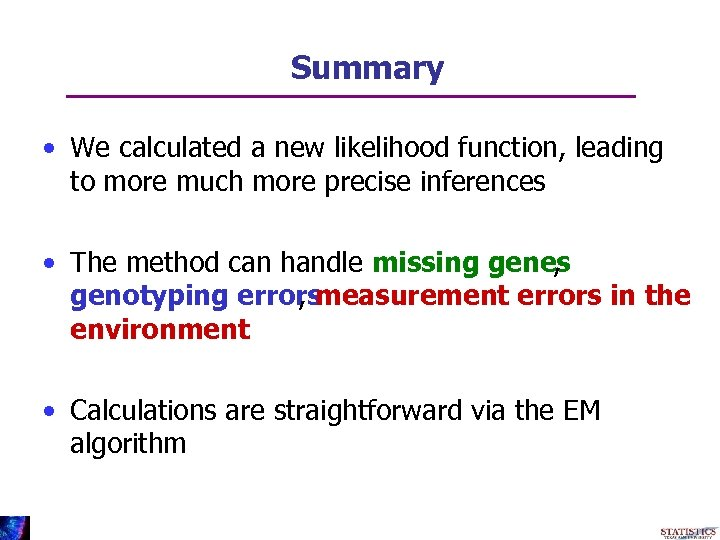Summary • We calculated a new likelihood function, leading to more much more precise inferences • The method can handle missing genes , genotyping errors , measurement errors in the environment • Calculations are straightforward via the EM algorithmSummary • Forced to face the dilemma • Lousy but robust method • Great but not robust method • We developed a fast, data adaptive, novel way of addressing this issue • In cases where one can predict the outcome, the EB method works as desired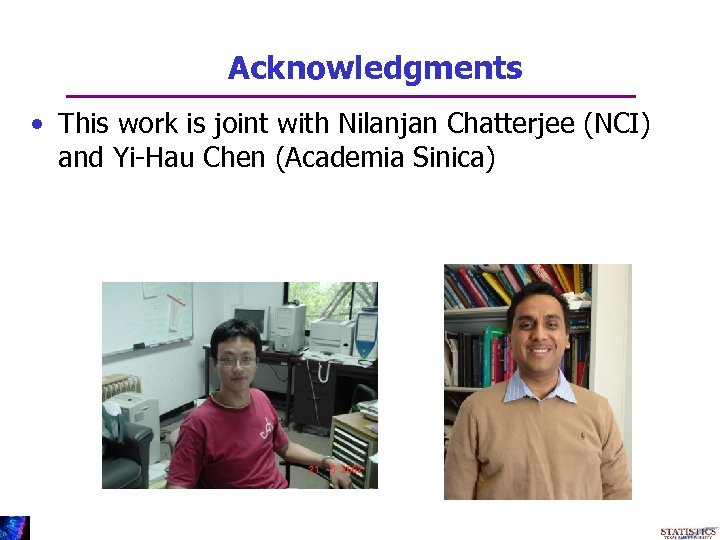Acknowledgments • This work is joint with Nilanjan Chatterjee (NCI) and Yi-Hau Chen (Academia Sinica)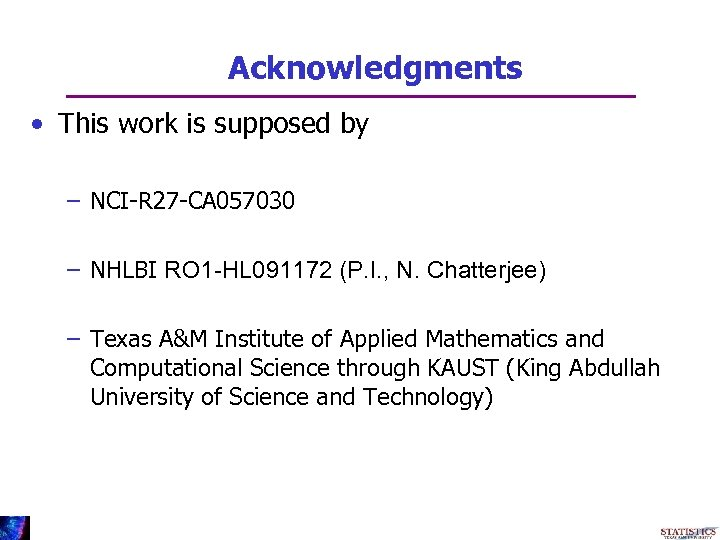Acknowledgments • This work is supposed by – NCI-R 27 -CA 057030 – NHLBI RO 1 -HL 091172 (P. I. , N. Chatterjee) – Texas A&M Institute of Applied Mathematics and Computational Science through KAUST (King Abdullah University of Science and Technology)